On Dark Matter Gravity Force Generated by the Expansion of the Universe

Delso J

Bachelor’s Degree in Physics by Zaragoza University, Spain

Article
Article Info
Author Info
Figures & Data

Abstract

Dark matter gravity force is generated by the expansion of the universe, gravitational force is not strong enough and an extra centripetal force is needed due to this expansion, this model also explains the high angular momentum of the Earth–Moon system, energy related to this new force is stored in the dark matter energy tensor D (4, 0) related to the Riemann curvature.

The cosmological redshift energy loss due to the expansion of the universe is stored in the dark matter energy tensor D (4, 0). The Cosmic Microwave Background energy loss has been stored in the dark matter energy tensor D (4, 0).

Dark matter gravity is generated by D(4,0) energy related directly to the S(4,0) tensor, however this gravity is attributed to exotic particles never detected, galaxies in our universe are rotating with such speed that the gravity generated by their observable matter could not possibly hold them together.

Total Energy T (4, 0) and energy tensors are defined to complete the General Relativity field equations. The Ricci decomposition is a way of breaking up the Riemann curvature tensor into three orthogonal tensors, Z (4, 0), Weyl tensor C (4, 0) and S (4, 0).

Index Terms

Dark Matter; Expansion of the Universe; Riemann Curvature; Earth Moon System; Cosmic Microwave Background Redshift; General Relativity Field Equations

Dark matter gravity force is generated by the expansion of the universe

In an expanding universe a satellite is in a uniform circular orbit around a planet, satellite mass m << planet mass M, initial radius r0, initial constant angular velocity ω0, initial velocity v0 << c, c the speed of light, due to the expansion of the universe the final radius rf = ar0, a > 1, final constant angular velocity ωf, initial gravitational force F0g equals centripetal force.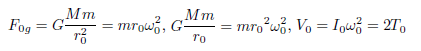In the expansion of the universe angles remain invariant, so ω0 = ωf and gravitational force Fg equals centripetal forceSince ω < ω0, gravitational force Fg is not strong enough and an extra centripetal force is needed, this extra force is attributed to exotic particles never detected.

Edm = Ef E0 = T0a2 − 2T0a1 + T0

Dark Matter energy Edm has been stored in the dark matter energy tensor D (4, 0) defined below, differentiating we get the dark matter force Fdm

Fdm = 2T0a + 2T0a−2

This model also explains the high angular momentum of the Earth Moon system .The definition of the dark matter energy leads to think that dark matter effect is zero in a newborn galaxy (a = 1) and is increasing when it is becoming older while the universe is expanding (increasing a). I suggest comparing observational data of dark matter effect for similar galaxies nowadays and in the past in order to confirm this equation.

Cosmological redshift energy loss due to the expansion of the universe

In an expanding closed universe, regardless our universe is closed or open, the final wave length of the photon when the closed universe reaches its maximum radius is .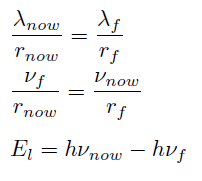where h is the Planck constant and El is the energy loss, in a closed universe after reaching its maximum expansion the universe comes backwards and will reach rnow again restoring the loss of energy to the photon, so this El has been stored and was not definitely lost.

The cosmological redshift energy loss due to the expansion of the universe is stored in the dark matter energy tensor D (4, 0) related directly to the S (4, 0) tensor; however this gravity is attributed to exotic particles never detected.

The Cosmic Microwave Background energy loss has been stored in the dark matter energy tensor D (4, 0); gravitational waves and other fields are subject to the same redshift phenomena.

Conformal energy U defined as a combination of C and the Hodge dual of C, dark matter energy D defined as a combination of S and the Hodge dual of S, similar definitions for V/Z and T/R

The Ricci decomposition is a way of breaking up the Riemann curvature tensor into three orthogonal tensors, Z , Weyl tensor C and S, S tensor generates the dark matter gravity   .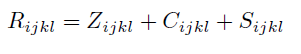where Rabcd is the Riemann tensor, Rab is the Ricci tensor, R is the Ricciscalar (the scalar curvature)

The conformal energy tensor U can be defined as a combination of C and the Hodge dual of C   .The new dark matter energy tensor D can be defined as a combination of S and the Hodge dual of SThe new energy tensor V can be defined as a combination of Z and the Hodge dual of Z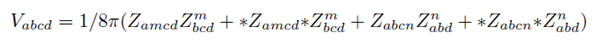The new Total Energy tensor T can be defined as a combination of the Riemann tensor R and the Hodge dual of RHodge Dual Definitions

The Hodge dual definition for Electromagnetic tensor and Weyl tensor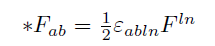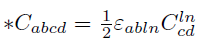The Hodge dual definition for dark matter S tensor, Z and R tensorsWeyl tensor C (4, 0) is related to the new Conformal Energy tensor U (4, 0). Dark matter tensor S (4, 0) is related to the new dark matter energy tensor D (4, 0). Z (4, 0) tensor is related to the new energy tensor V (4, 0). Riemann tensor R (4, 0) is related to the new Total Energy tensor T (4, 0).

Complete General Relativity Field Equations

The complete field equations are described by a new T(4,0) tensor for Total Energy, the new conformal energy tensor U(4,0), the new energy tensor V(4,0) and the new dark matter energy tensor D(4,0).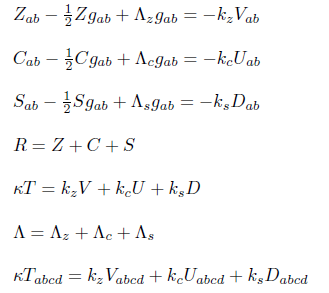In the general theory of relativity the Einstein field equations relate the geometry of spacetime to the distribution of matter .References

1. Jesus Delso Lapuerta (2020) On the conformal energy tensor defined as a combination of Weyl tensor and the Hodge dual of Weyl tensor. Vixra.org Relativity and Cosmology 2-3.
2. Roger Penrose (2006) El camino a la realidad. Random House Mondadori, Barcelona 619.
3. Roger Penrose (2006) El camino a la realidad. Random House Mondadori, Barcelona 629.
4. Roger Penrose (2006) El camino a la realidad. Random House Mondadori, Barcelona 1255.
5. Albert Einstein (1985) El significado de la relatividad Planeta-De Agostini, Barcelona 175.
6. Frank J Tipler (1996) La Fsica de la inmortalidad. Alianza Editorial, Madrid 572.
7. Stevenson DJ (1987) Origin of the moon the collision hypothesis. Annual Review of Earth and Planetary Sciences. 15: 271-315.
8. Besse Arthur L (1987) Einstein manifolds, Ergebnisse der Mathematik undihrer Grenzgebiete Results in Mathematics and Related Areas 3:10.
9. Sharpe RW (1997) Differential Geometry: Cartan's Generalization of Klein's Erlangen Program, Springer-Verlag, New York. Section 6.1 discusses the decomposition. Versions of the decomposition also enter into the discussion of conformal and projective geometries, in chapters 7 and 8.
10. Singer IM, Thorpe JA (1969) The curvature of 4-dimensional Einstein spaces, Global Analysis (Papers in Honor of K. Kodaira), Univ. Tokyo Press 355-365.

Review Article

Publication history

Accepted date: March 29, 2021
Published date: April 09, 2021

©2020 Delso J. This is an open-access article distributed under the terms of the Creative Commons Attribution License, which permits unrestricted use, distribution, and reproduction in any medium, provided the original author and source are credited.

Citation

Delso J. (2020) On Dark Matter Gravity Force Generated by the Expansion of the Universe. OSP J Phy Astronomy 2: JPA-2-111

Jesus Delso Lapuerta

Bachelor's Degree in Physics by Zaragoza University, Spain.
jesus.delso@gmail.com

No Figures